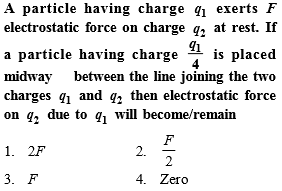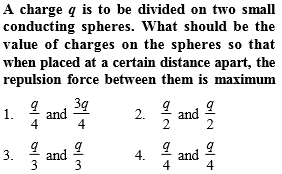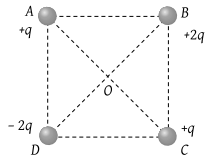You’ve reached the end of your free Videos limit.
#2 | Basic Properties of Charge
(Physics) > Electric Charges and Fields
Related Practice Questions :Complete Question Bank + Test Series
Complete Question Bank

Difficulty Level:Complete Question Bank + Test Series
Complete Question Bank

Difficulty Level:

The law, governing the force between electric charges is known as

(1) Ampere's law

(2) Ohm's law

(4) Coulomb's law

Complete Question Bank + Test Series
Complete Question Bank

Difficulty Level:

A soap bubble is given a negative charge, then its radius

(1) Decreases

(2) Increases

(3) Remains unchanged

(4) Nothing can be predicted as information is insufficient

Complete Question Bank + Test Series
Complete Question Bank

Difficulty Level:

Four charges are arranged at the corners of a square ABCD, as shown in the adjoining figure. The force on the charge kept at the centre O is(1) Zero

(2) Along the diagonal AC

(3) Along the diagonal BD

(4) Perpendicular to side AB